# Brook’s Theorem

• Difficulty Level : Expert
• Last Updated : 25 Mar, 2022

Brook’s Theorem is one of the most well-known graph coloring theorems. Graph coloring is a subset of graph labeling, in graph theory. It involves the assignment of labels to elements of a graph, commonly referred to as “colors,” according to specific constraints. In its most basic form, vertex coloring is a method of coloring the vertices of a graph so that no two neighboring vertices are the same color. Now, let’s take a look at how Brook’s Theorem helps with graph coloring.

### Statement of Brook’s theorem

In Graph Theory, Brook’s Theorem illustrates the relationship between a graph’s maximum degree and its chromatic number. Brook’s Theorem states that:

If G is a connected simple graph and is neither an odd cycle nor a complete graph i.e. χ(G)≥3 then

χ(G) ≤ k, where k denotes the maximum degree of G and χ(G) denotes the chromatic number of G.

• An odd cycle means a cycle with an odd length, that is, with an odd number of edges.
• A complete graph is a graph in which a vertex should have edges with all other vertices.
• A graph’s chromatic number is the smallest number of colors required to color the vertices so that no two neighboring vertices have the same color.
• The biggest number of neighbors of a vertex in graph G is its maximum degree.

Brooks’ Theorem extends this assertion of the Greedy Algorithm which shows that χ(G) ≤ k +1, for any graph G. For example,Fig. 1: Graph satisfying brook’s theorem

As you can see in fig. 1, the chromatic number of the graph i.e. χ(G) is 3 & the maximum degree i.e. k  is 3.  So the χ(G) = k, satisfying Brook’s theorem.

### Proof of Brook’s theorem

Let’s say k = (G). G is either a cycle or a path if k = 2. We can suppose that k≥3. We’ll design an ordering in which each vertex has a maximum of k-1 vertices preceding it.

• Let us first assume that there is a vertex V with the value d(v)<k. Find a T spanning tree of G and root it at v. Put v as and the other vertices are ordered in T in such a way that all vertices at distance are before all vertices at distance i+ 1 from v. In this arrangement, every vertex has at most k-1 vertices preceding it.
• Assume G has a cut vertex v and is k-regular. Consider the components G1,…, Gl, with 2≤l of G′ equal G – v. Each of G – i’s vertex vi has the value VVi ∈ E(G), therefore dGi(vi) = k – 1. We can appropriately k-color each of Gi‘s using the previous way. In reality, we can tint Gi so that the color of vi is 1, if necessary, by swapping the colors.
• Assume G is 2-connected and k-regular.
• Assume that there is a vertex v with two neighbors V1, V2 and that V1, V2 ∉ E and  G − {V1, V2} are connected. Find a spanning tree of  G − {V1, V2} with a root in v and order it the same way you did before. V1, V2 should be added at the beginning of the ordering. Greedy Coloring will then give both V1 and V2 the same color. Every vertex w ≠ v has a maximum of k-1 neighbors in front of it. Vertex v has k but V1, V2 have the same color and therefore we can give a color to v.
• We shall now demonstrate that such a triple v, v1, v2 exists at all times. Choose an arbitrary vertex x. If κ(G − x) ≥ 2 is true, then v1 = x and v2 is a vertex at a distance of two times x. There must be such a vertex, because if there is an edge from x to every vertex from G−x, then G is complete because G is k-regular. Finally, v1 and v2 have a common neighbour in v. Additionally, G − {v1, v2} = G − {x, v2} is related.
• Let κ(G − x) = 1 be the cut vertex of  G − x, and w be the cut vertex of G−x.
• Let H represent a block of G−x that is a leaf in the block graph, and a represent the cut vertex of G−x which is written in H. Then x has a different neighbor in H than a because otherwise, G-a is unconnected.
• Let v = x be the neighbors of x from two different leaf blocks of G-x, and v1, v2 be the neighbors of x from two different leaf blocks of G−x. There is clearly no connection between v1 and v2, and G − {v1, v2} is connected as d(x) ≥ 3.

### Sample Problems

Question 1: Prove Brook’s theorem for the given graph.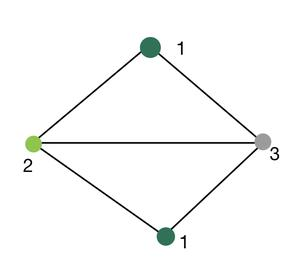Solution:

The above graph,

The chromatic number of the graph, i.e. χ(G) = 3.

The maximum degree of the graph i.e. k = 3.

Therefore, χ(G) = k. Thus, the above graph proves Brook’s Theorem.

Question 2: Prove Brook’s theorem for the given graph.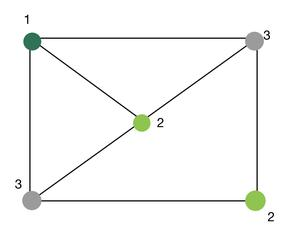Solution:

The above graph,

The chromatic number of the graph, i.e. χ(G) = 3.

The maximum degree of the graph i.e. k = 3.

Therefore, χ(G) = k. Thus, the above graph proves Brook’s Theorem.

Question 3: Prove Brook’s theorem for the given graph.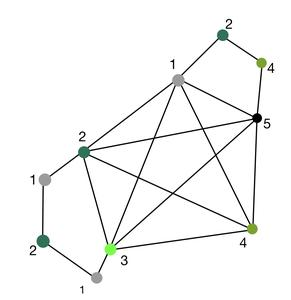Solution:

The above graph,

The chromatic number of the graph, i.e. χ(G) = 5.

The maximum degree of the graph i.e. k = 5.

Therefore, χ(G) = k. Thus, the above graph proves Brook’s Theorem.

Question 4: Prove Brook’s theorem for the given graph.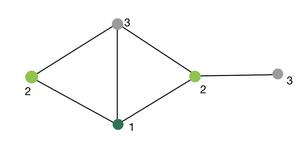Solution:

The above graph,

The chromatic number of the graph, i.e. χ(G) = 3.

The maximum degree of the graph i.e. k = 3.

Therefore, χ(G) = k. Thus, the above graph proves Brook’s Theorem.

Question 5: Prove Brook’s theorem for the given graph.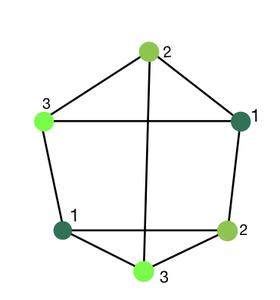Solution:

The above graph,

The chromatic number of the graph, i.e. χ(G) = 3.

The maximum degree of the graph i.e. k = 3.

Therefore, χ(G) = k. Thus, the above graph proves Brook’s Theorem.

Question 6: Prove Brook’s theorem for the given graph.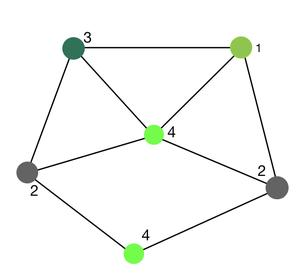Solution:

The above graph,

The chromatic number of the graph, i.e. χ(G) = 4.

The maximum degree of the graph i.e. k = 4.

Therefore, χ(G) = k. Thus, the above graph proves Brook’s Theorem.

My Personal Notes arrow_drop_up